4679 字

# 诺谟图

## 原理篇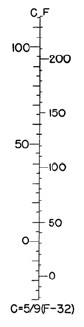$$f_1(u) + f_2(v) = f_3(w)$$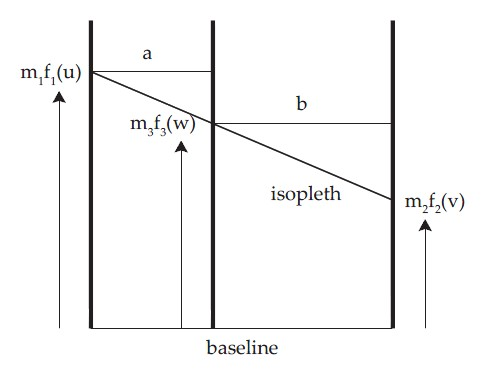$$\frac{m_1f_1(u)-m_3f_3(w)}{a} = \frac{m_3f_3(w) - m_2f_2(v)}{b}$$

$$m_1f_1(u) + \frac{a}{b} m_2f_2(v) = (1+ \frac{a}{b})m_3f_3(w)$$

$$m_1 = \frac{a}{b}m_2 = (1+ \frac{a}{b})m_3$$

$$\frac{m_1}{m_2}=\frac{a}{b}$$

$$m_3 = \frac{m_1*m_2}{m_1+m_2}$$

$$log(cd) = log c + log d$$

$$log(c^d) = d log c$$

$$(1.2*D + 0.47)^{0.68}*(0.91T)^{3/2} = N$$

$$0.68 log(1.2D + 0.47) + 1.5 log T = logN − 1.5 log 0.91$$

$$m_1 =\frac{11}{0.68 log(1.2(8.0) + 0.47) − 0.68 log(1.2(1.0) + 0.47)}= 20.73$$

$$m_2 =\frac{11}{1.5 log 2.0 − 1.5 log 1.0}= 24.36$$

$$m_3 = \frac{m_1*m_2}{m_1 +m_2}= 11.20$$

$$\frac{a}{b}= \frac{m_1}{m_2}= 0.851$$

$$a + b = 6$$

$$b = 3.241 cm, a = 2.759 cm$$

$$20.73 *(0.68 log(1.2D + 0.47) − 0.68 log(1.2(1.0) + 0.47))$$

$$24.36(1.5 log T − 1.5 log 1.0)$$

$$(1.2(1.0) + 0.47)^{0.68}*(0.91(1.0))^{1.5} = 1.230$$

$$11.20*(logN − 1.5 log(0.91))$$

OK,现在我们可以得到这张诺谟图了。使用的时候我们只需要知道任意两轴上的数做延长或相交，就可以知道第三轴的数了。这个过程完全依赖直尺比划就可以，类似于查表（不知道现在还教不教）。我们可以看出诺谟图在求解复杂方程上是很方便的，甚至可以求解一些无解析解的方程。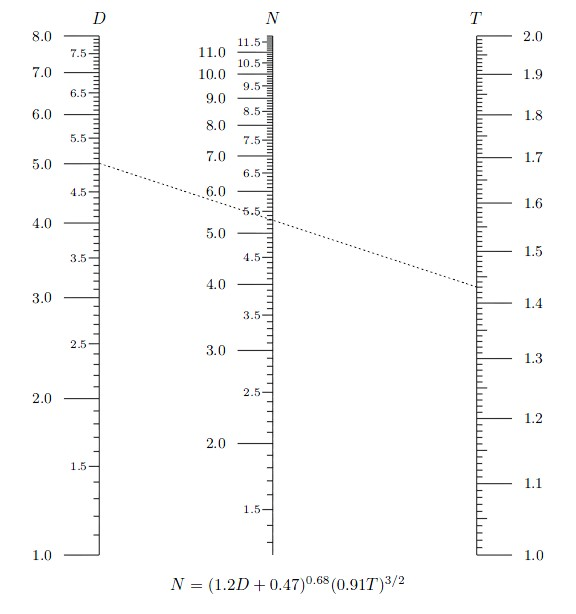$$f_3(w) = \frac{f_1(u)}{f_2(v)}$$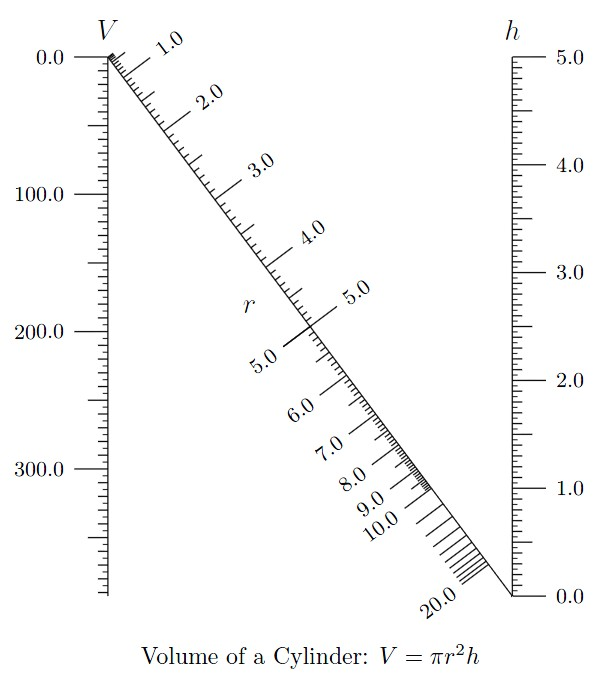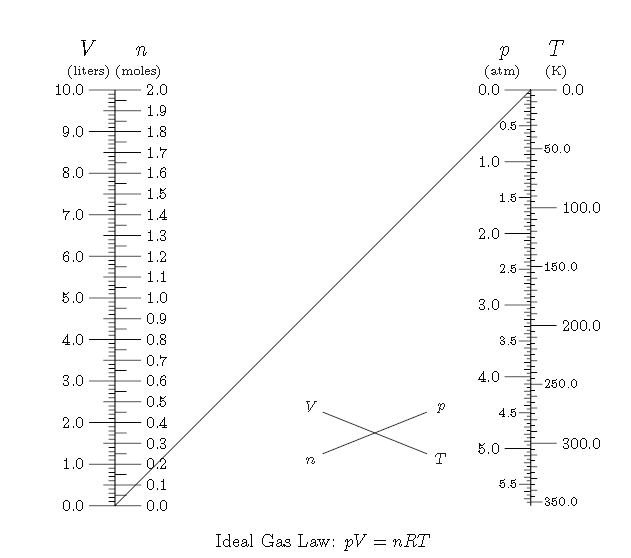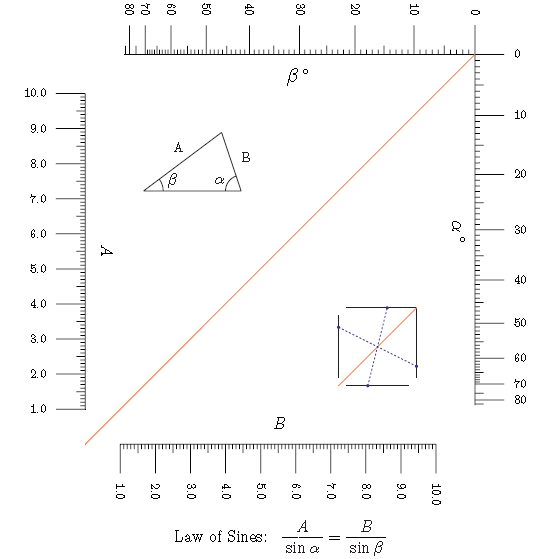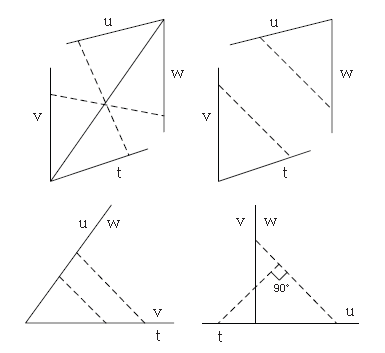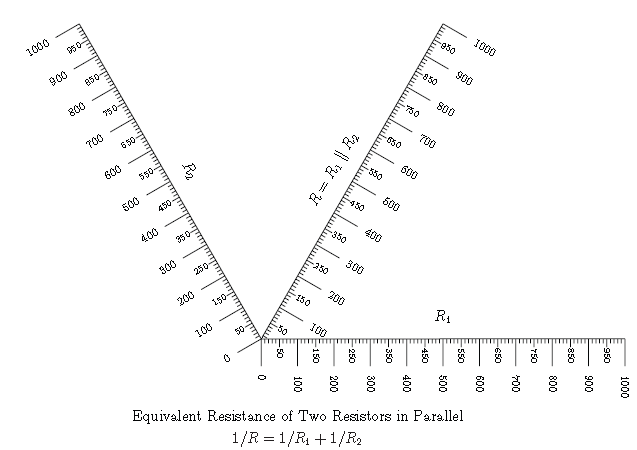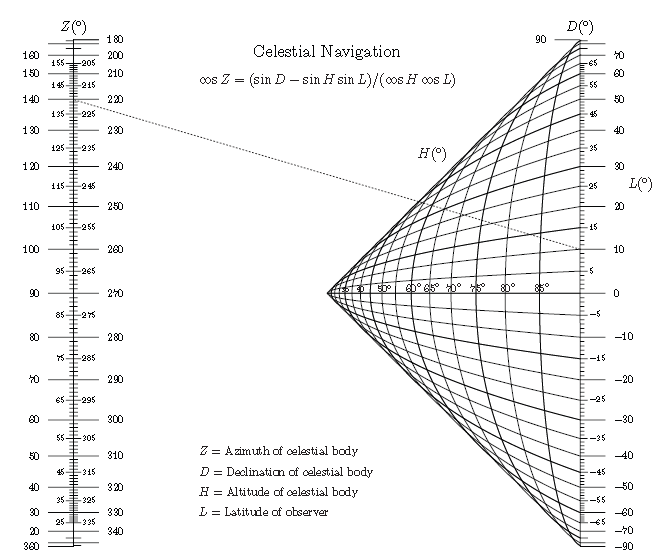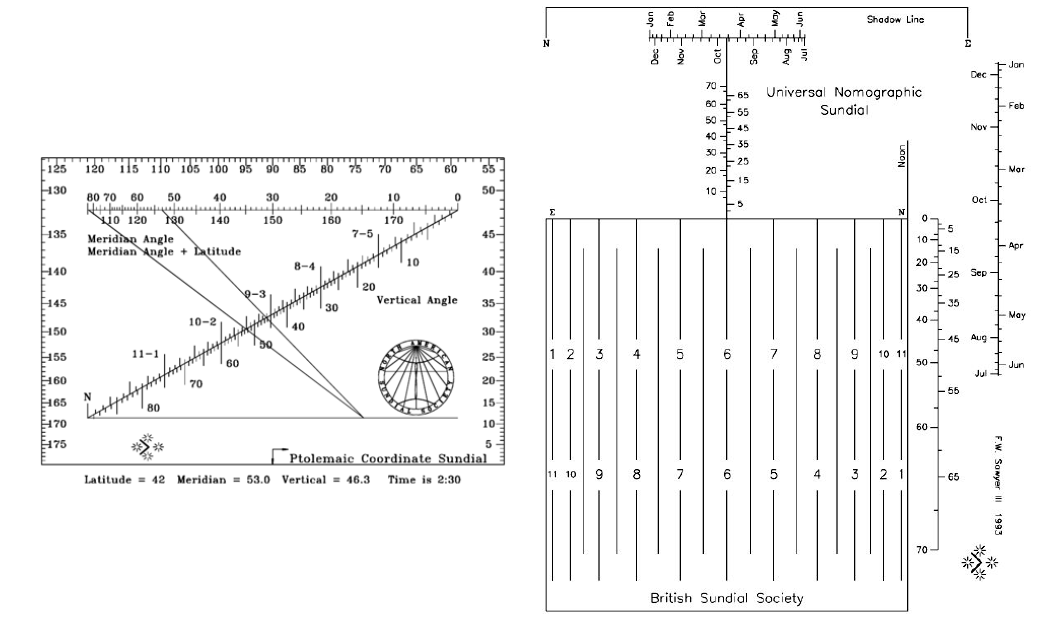## 历史篇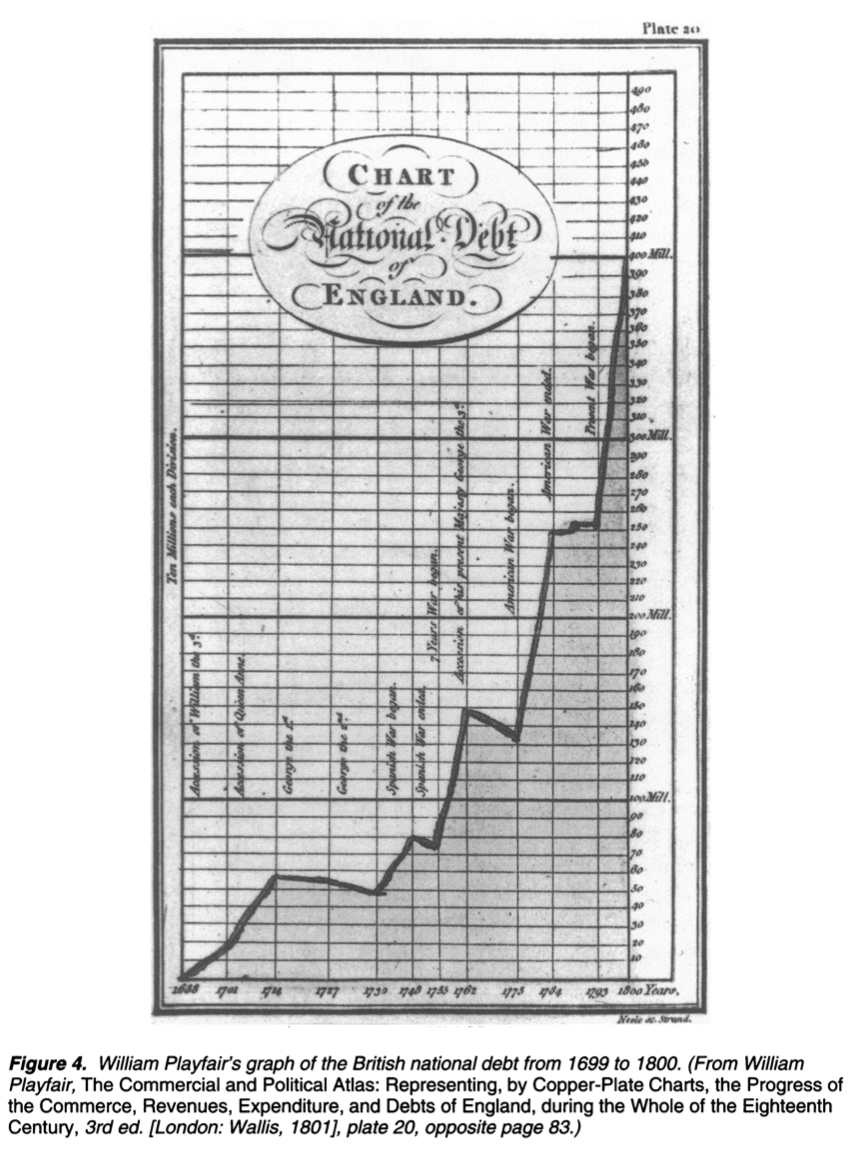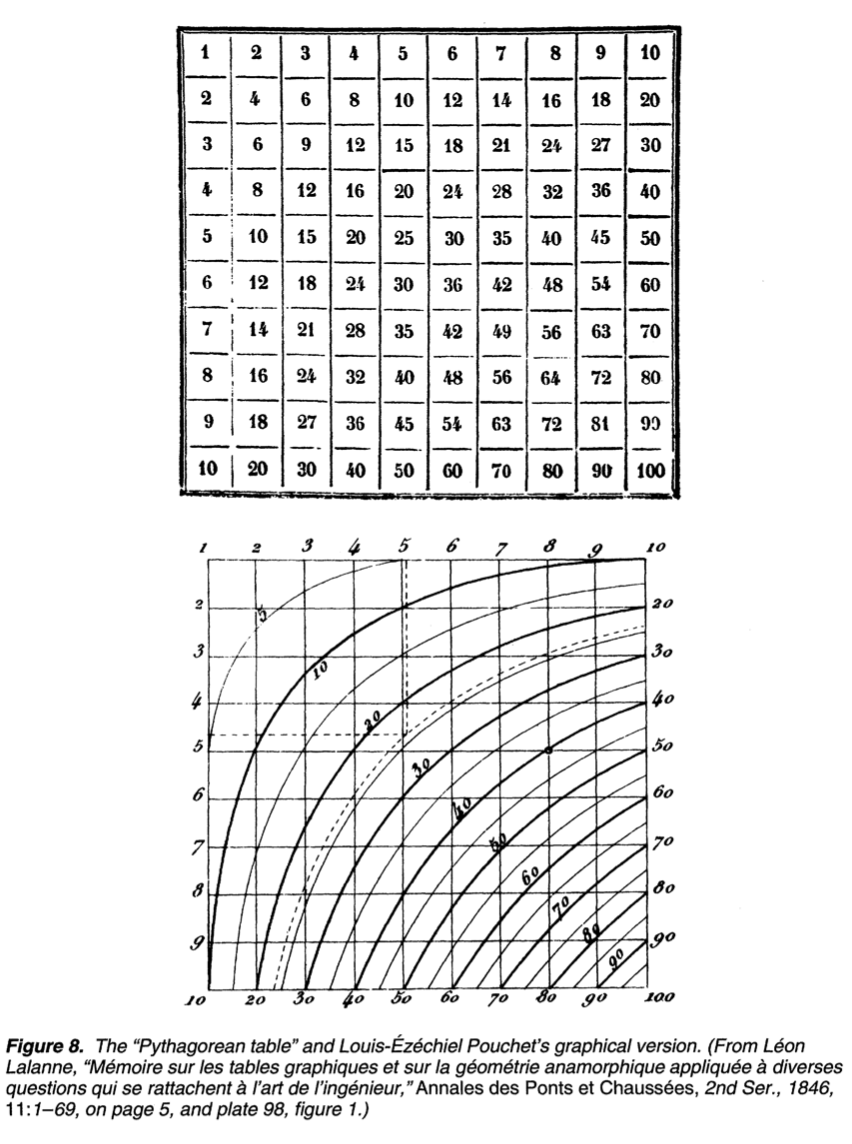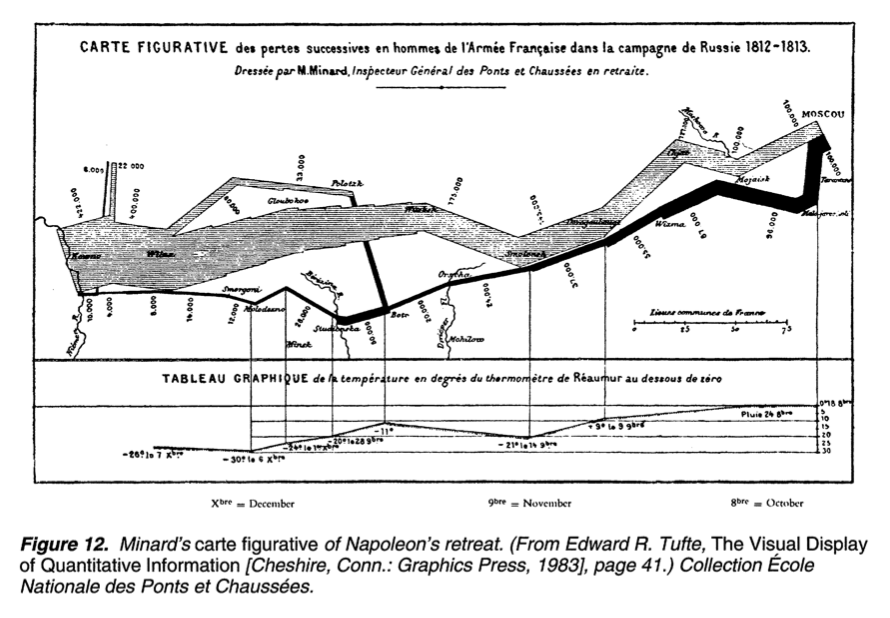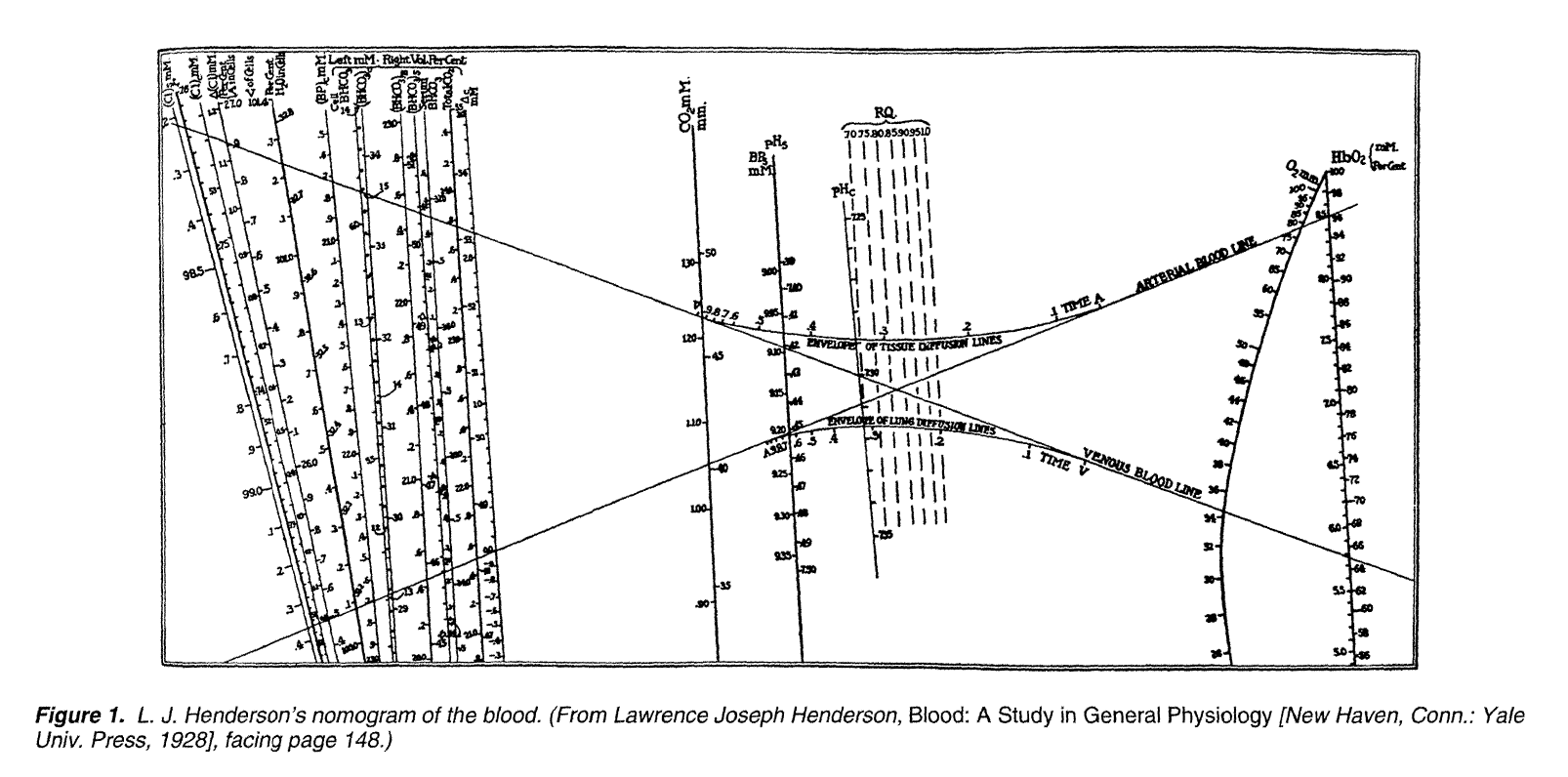## 回归篇